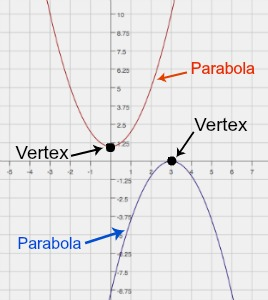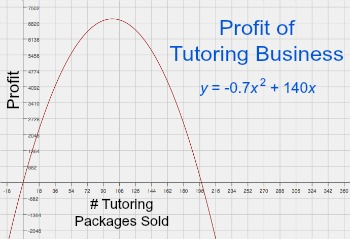# How to Find the Vertex of a Quadratic Equation

An error occurred trying to load this video.

Try refreshing the page, or contact customer support.

Coming up next: Functions: Identification, Notation & Practice Problems

### You're on a roll. Keep up the good work!

Replay
Your next lesson will play in 10 seconds
• 0:04 Steps to Solve
• 1:27 Solution
• 2:06 Application
• 4:08 Lesson Summary

Want to watch this again later?

Timeline
Autoplay
Autoplay
Speed

#### Recommended Lessons and Courses for You

Lesson Transcript
Instructor: Laura Pennington

Laura has taught collegiate mathematics and holds a master's degree in pure mathematics.

This lesson will show how to find the vertex of a quadratic equation. We will also see why this process is so useful to know and apply it to a real world application that could easily come up in one's life.

## Steps to Solve

We are going to learn how to find the vertex of a quadratic equation. As you may know, the graph of a quadratic equation, y = ax2 + bx + c, is the shape of a parabola. A parabola looks like a U or an upside-down U. The vertex of a quadratic equation is the maximum or minimum point on the equation's parabola.See why this point is so important? It's always incredibly useful to know the maximum or minimum value of an equation, especially when that equation represents real world phenomena. What's really nice about the vertex of a quadratic equation is that it's pretty easy to find. To do so, we use the following steps:

1. Get the equation in the form y = ax2 + bx + c.
2. Calculate -b / 2a. This is the x-coordinate of the vertex.
3. To find the y-coordinate of the vertex, simply plug the value of -b / 2a into the equation for x and solve for y. This is the y-coordinate of the vertex.

After following these steps, you have your x and y coordinates of the vertex, so you have your vertex. Not too bad, huh? We see that the vertex of a quadratic equation, y = ax2 + bx + c, is the following point.

(-b / 2a, a(-b / 2a)2 + b(-b / 2a) + c)

## Solution

To find the vertex of a quadratic equation, y = ax2 + bx + c, we find the point (-b / 2a, a(-b / 2a)2 + b(-b / 2a) + c), by following these steps.

1. Get the equation in the form y = ax2 + bx + c.
2. Calculate -b / 2a. This is the x-coordinate of the vertex.
3. To find the y-coordinate of the vertex, simply plug the value of -b / 2a into the equation for x and solve for y. This is the y-coordinate of the vertex.

## Application

We saw that this process doesn't look so bad, so let's go ahead and give it a go with an application. Suppose you and a few of your friends decide to open a math tutoring business. Taking time and travel expenses into account, your business's monthly profit can be modeled by the quadratic equation:

y = -0.7x2 + 140x

where y is the profit when you sell x tutoring packages. We see that this is the quadratic equation, and its graph is shown here:Now, when it comes to the profit of your business, what is the number one question you want to know about it? It's what is the maximum amount of profit you can make, and how many tutoring packages do you have to sell to get that maximum profit?

To unlock this lesson you must be a Study.com Member.

### Register to view this lesson

Are you a student or a teacher?

### Unlock Your Education

#### See for yourself why 30 million people use Study.com

##### Become a Study.com member and start learning now.
Back
What teachers are saying about Study.com

### Earning College Credit

Did you know… We have over 160 college courses that prepare you to earn credit by exam that is accepted by over 1,500 colleges and universities. You can test out of the first two years of college and save thousands off your degree. Anyone can earn credit-by-exam regardless of age or education level.